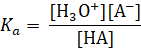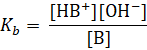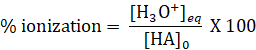It looks like you're using Internet Explorer 11 or older. This website works best with modern browsers such as the latest versions of Chrome, Firefox, Safari, and Edge. If you continue with this browser, you may see unexpected results.

# Chemistry

Brønsted-Lowry Acids and Bases

Brønsted-Lowry acid is defined as a compound that donates a proton to another compound and known as a proton donor

Brønsted-Lowry base is the compound that accepts a proton from another compound and known as a proton acceptor

Example 1

In the chemical equation below identify the Brønsted-Lowry acid.

C6H5OH + NH2 → C6H5O + NH3

C6H5OH is losing an H+ ion thus donating a proton. Based on the definition above it is a Brønsted-Lowry acid. NH2−  is accepting the Htherefore it is a proton acceptor and can be concluded as a base using the definition for Brønsted-Lowry base.

Amphiprotic, or amphoteric species: Species that can either accept or donate protons.An example is shown in the figure below where bicarbonate and water can either be proton donor (acid) or acceptor (base), thus they both are amphiprotic.The pH Scale

pH refers to the power of the hydronium ion which measured through the concentration of (hydronium) H3O+ ions. In other words pH scale helps to measure how acidic or basic a solution is. Lower the value of pH the more acidic a solution becomes. For example a solution with pH two is more acidic than a solution with pH of four. Neutral solution such as water (at 25 °C) has a pH value of seven, and basic solution has a pH that's greater than seven.

pH is calculated using the following formula:

pH = -log [ H3O+ ]

If the pH of a solution is given then the hydronium ion concentration can be calculated using the following formula:

H3O+ ] = 10-pH

Example 1:

Find the pH of pure water if it has the hydronium ion concentration of 1 X 10-7 M ?

H3O+ ] = 1 X 10-7 M

pH = -log [ 1 X 10-7 ] = -(-7.00) = 7.00

Pure water is a neutral solution therefore its pH is 7 at 25 °C.

Example 2:

Calculate the hydronium ion concentration of a solution if it has a pH of  7.3

H3O+ ] = 10-pH

H3O+ ] = 10-7.3

H3O+ ] = 5.0 x 10-8 M

The pOH Scale

Similarly the hydroxide ion concentration of a solution can be expressed using the pOH scale.

The following formula is used to calculate the pOH:

pOH = -log [ OH- ]

If the pOH of a solution is given then the hydroxide ion concentration can be calculated using the following formula:

[ OH- ] = 10-pOH

Example 3:

Calculate the pOH of a solution that has the hydroxide ion concentration of 1.25 x 10-6 M.

pOH = -log [ OH- ] = pOH = -log [ 1.25 x 10-6] = -(5.90) = 5.90

Example 4:

Calculate the hydroxide ion concentration of a solution that has a pOH of 4.37

[ OH- ] = 10-pOH =[ OH- ] = 10-4.37 4.27 x 10-5 M

The Relationship Between pH and pOH

At 25 °C a water solution's pH value and pOH value are related as shown below:

pH + pOH = 14

Example 5:

Calculate the pH of a solution that has a pOH of 11.75

pH =14 - pOH = 14 - 11.75 = 2.25Figure 1: The pH scale. From Vecteezy.com (n.d.). Retrieved April 13, 2021 from https://www.vecteezy.com/free-vector/ph-scale

Relative Strengths of Acids and Bases

Relative strengths of acids and bases characterize how well an acid or base ionizes when dissolved in water. It can be quantized using the acid (Ka), base (Kb) ionization constants, or percent ionization.

Strong acids and bases: Strong acids or bases have 100% ionization in water

Examples of strong acids:

• Nitric acid (HNO3)
• Sulfuric acid (H2SO4)
• Perchloric acid (HClO4)
• Hydrochloric acid (HCl)
• Hydrobromic acid (HBr)
• Hydroiodic acid (HI)

Examples of strong bases:

• Sodium hydroxide (NaOH)
• Calcium hydroxide (Ca(OH)2)
• Lithium hydroxide (LiOH)
• Potassium hydroxide (KOH)
• Strontium hydroxide (Sr(OH)2)
• Barium hydroxide (Ba(OH)2)

Weak acids and bases: They do not dissociate completely in the solution therefore the reaction is usually written as a reversible reaction

Conjugate base: Conjugate base is what remains after an acid donates a proton (H+) because it can now act as a proton acceptor (base) in the reverse reaction.

Conjugate acid: When a base accepts an H+ it becomes a conjugate acid because it can now donate a proton (H+)Figure 2: Conjugate acid-base pairs. From LibreTexts (2019, August 22), Buffers and conjugate acid-base pairs. Chemistry. https://chem.libretexts.org/Courses/Sacramento_City_College/SCC%3A_CHEM_330_-_Adventures_in_Chemistry_(Alviar-Agnew)/07%3A_Acids_and_Bases/7.07%3A_Buffers_and_Conjugate_Acid-Base_Pairs

Acid ionization constant

The acid ionization constant can be calculated using the following equation for a reaction of acid HA.

HA(aq) + H2O(l) ⇌ H3O+(aq) + A(aq)Base ionization constant

The base ionization constant can be calculated using the following equation for a reaction of base HB.

B(aq) + H2O(l) ⇌ HB+(aq) + OH(aq)Percent Ionization

The ratio of the concentration of ionized weak acid to its initial acid concentration is known as percent ionization. It's another way of measuring the strength of weak acids and calculated using the following equation.Mathematical product of conjugate acid-base pair is equal to KwAdding the above two equation gives the following:Photo credit: Openstax

With that the Kw becomes:Photo credit: Openstax

Polyprotic Acids

There are two different types of acids based on the number of protons they can give up:

Monoprotic acid: An acid that can only donate one proton.

Example: HCl, HNO3, HCN

Diprotic acid: An acid that consists of two hydrogen atoms and can donate two protons (hydrogen atoms).

Example: H2SO4

First ionization: H2SO4(aq) + H2O(l) H3O+(aq) + HSO4-(aq)          Ka1 = more than 102

Second ionization: HSO4-(aq) H2O(l) H3O+(aq)  + SO42-(aq)         Ka2 = 1.2 X 10-2

Triprotic acid: This one contains three hydrogen atoms and can donate three protons.

Example: H3PO4

First ionization: H3PO4(aq) + H2O(l) ⇌ H3O+(aq) + H2PO4-(aq)           Ka1 = 7.5 × 103

Second ionization: H2PO4-(aq) + H2O(l) ⇌ H3O+(aq) + HPO42−(aq)    Ka2 = 6.2 × 108

Third ionization: HPO42−(aq) + H2O(l) ⇌ H3O+(aq) + PO43−(aq)         Ka3 = 4.2 × 1013